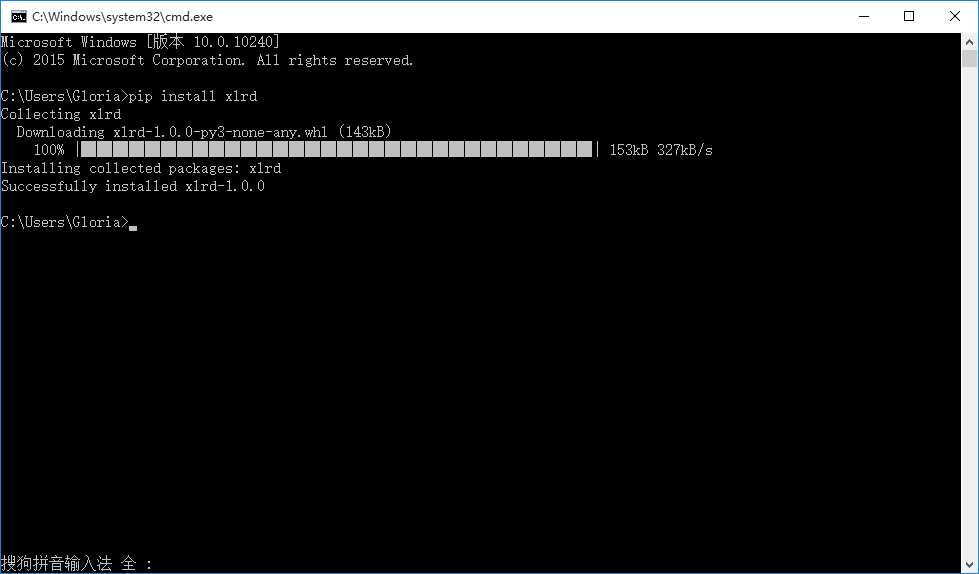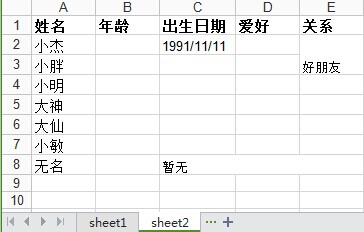# python中使用xlrd、xlwt操作excel表格详解_python

import xlrd data=xlrd.open_workbook('E:\\\\成绩表.xls') table=data.sheets() l=[] for i in range(table.nrows): l.append(table.row_values(i))这样sheet1的内容就都在名为l的list里了python操作excel主要用到xlrd和xlwt这两个库，即xlrd是读excel，xlwt是写excel的库。可从这里下载https://pypi.python.org/pypi。下面分别记录python读和写excel.

2种做法： 第一种用com import win32com.client from win32com.client import constants f=r\"h:\\Python\\Examples\\test.xls DELETE_THIS=\"X exc=win32com.client.gencache.EnsureDispatch(\"Excel.python读excel――xlrd1、使用xlrd的xlrd.open_workbook()方法打开文件返回的是一个只读对象，只能读Excel内容，不能向Excel写入数据；2、使用xlwt的xlwt.Workbook()方法是创建一个空的Excel对象，然后向里面写入;所以读取和python 对 excel基本的操作如下：

# -*- coding: utf-8 -*-import xlrdimport xlwtfrom datetime import date,datetimedef read_excel(): # 打开文件 workbook = xlrd.open_workbook(r'F:\demo.xlsx') # 获取所有sheet print workbook.sheet_names() # [u'sheet1', u'sheet2'] sheet2_name = workbook.sheet_names() # 根据sheet索引或者名称获取sheet内容 sheet2 = workbook.sheet_by_index(1) # sheet索引从0开始 sheet2 = workbook.sheet_by_name('sheet2') # sheet的名称，行数，列数 print sheet2.name,sheet2.nrows,sheet2.ncols # 获取整行和整列的值（数组） rows = sheet2.row_values(3) # 获取第四行内容 cols = sheet2.col_values(2) # 获取第三列内容 print rows print cols # 获取单元格内容 print sheet2.cell(1,0).value.encode('utf-8') print sheet2.cell_value(1,0).encode('utf-8') print sheet2.row(1).value.encode('utf-8') # 获取单元格内容的数据类型 print sheet2.cell(1,0).ctypeif __name__ == '__main__': read_excel()1、python读取excel中单元格内容为日期的方式

python读取excel中单元格的内容返回的有5种类型，即上面例子中的ctype:

ctype : 0 empty,1 string, 2 number, 3 date, 4 boolean, 5 error

>>> sheet2.cell(2,2).ctype #1990/2/22>>> sheet2.cell(2,1).ctype #24>>> sheet2.cell(2,0).ctype #小胖>>> sheet2.cell(2,4).ctype #空值（这里是合并单元格的原因）>>> sheet2.cell(2,2).value #1990/2/2233656.0>>> xlrd.xldate_as_tuple(sheet2.cell_value(2,2),workbook.datemode)(1992, 2, 22, 0, 0, 0)>>> date_value = xlrd.xldate_as_tuple(sheet2.cell_value(2,2),workbook.datemode)>>> date_value(1992, 2, 22, 0, 0, 0)>>> date(*date_value[:3])datetime.date(1992, 2, 22)>>> date(*date_value[:3]).strftime('%Y/%m/%d') '1992/02/22'

if (sheet.cell(row,col).ctype == 3): date_value = xlrd.xldate_as_tuple(sheet.cell_value(rows,3),book.datemode) date_tmp = date(*date_value[:3]).strftime('%Y/%m/%d')

>>> sheet2.col_values(4)[u'\u5173\u7cfb', u'\u597d\u670b\u53cb', '', u'\u540c\u5b66', '', '', u'\u4e00\u4e2a\u4eba', '']>>> for i in range(sheet2.nrows): print sheet2.col_values(4)[i]关系好朋友同学一个人>>> sheet2.row_values(7)[u'\u65e0\u540d', 20.0, u'\u6682\u65e0', '', '']>>> for i in range(sheet2.ncols): print sheet2.row_values(7)[i]无名20.0暂无>>>

2、读取合并单元格的内容

>>> print sheet2.col_values(4)好朋友>>> print sheet2.row_values(7)暂无　>>> sheet2.merged_cells # 明明有合并的单元格，为何这里是空 []

3、获取合并的单元格

>>> workbook = xlrd.open_workbook(r'F:\demo.xlsx',formatting_info=True)>>> sheet2 = workbook.sheet_by_name('sheet2')>>> sheet2.merged_cells[(7, 8, 2, 5), (1, 3, 4, 5), (3, 6, 4, 5)]

merged_cells返回的这四个参数的含义是：(row,row_range,col,col_range),其中[row,row_range)包括row,不包括row_range,col也是一样，即(1, 3, 4, 5)的含义是：第1到2行（不包括3）合并，(7, 8, 2, 5)的含义是：第2到4列合并。

>>> print sheet2.cell_value(1,4) #(1, 3, 4, 5)好朋友>>> print sheet2.cell_value(3,4) #(3, 6, 4, 5)同学>>> print sheet2.cell_value(7,2) #(7, 8, 2, 5)暂无

>>> merge = []>>> for (rlow,rhigh,clow,chigh) in sheet2.merged_cells: merge.append([rlow,clow]) >>> merge[[7, 2], [1, 4], [3, 4]]>>> for index in merge: print sheet2.cell_value(index,index) 暂无好朋友同学>>>

python写excel――xlwt'''设置单元格样式'''def set_style(name,height,bold=False): style = xlwt.XFStyle() # 初始化样式 font = xlwt.Font() # 为样式创建字体 font.name = name # 'Times New Roman' font.bold = bold font.color_index = 4 font.height = height # borders= xlwt.Borders() # borders.left= 6 # borders.right= 6 # borders.top= 6 # borders.bottom= 6 style.font = font # style.borders = borders return style#写exceldef write_excel(): f = xlwt.Workbook() #创建工作簿 ''' 创建第一个sheet: sheet1 ''' sheet1 = f.add_sheet(u'sheet1',cell_overwrite_ok=True) #创建sheet row0 = [u'业务',u'状态',u'北京',u'上海',u'广州',u'深圳',u'状态小计',u'合计'] column0 = [u'机票',u'船票',u'火车票',u'汽车票',u'其它'] status = [u'预订',u'出票',u'退票',u'业务小计'] #生成第一行 for i in range(0,len(row0)): sheet1.write(0,i,row0[i],set_style('Times New Roman',220,True)) #生成第一列和最后一列(合并4行) i, j = 1, 0 while i < 4*len(column0) and j < len(column0): sheet1.write_merge(i,i+3,0,0,column0[j],set_style('Arial',220,True)) #第一列 sheet1.write_merge(i,i+3,7,7) #最后一列"合计" i += 4 j += 1 sheet1.write_merge(21,21,0,1,u'合计',set_style('Times New Roman',220,True)) #生成第二列 i = 0 while i < 4*len(column0): for j in range(0,len(status)): sheet1.write(j+i+1,1,status[j]) i += 4 f.save('demo1.xlsx') #保存文件if __name__ == '__main__': #generate_workbook() #read_excel() write_excel()

write_merge(x, x + m, y, w + n, string, sytle)

x表示行，y表示列，m表示跨行个数，n表示跨列个数，string表示要写入的单元格内容，style表示单元格样式。其中，x，y，w，h，都是以0开始计算的。''' 创建第二个sheet: sheet2 ''' sheet2 = f.add_sheet(u'sheet2',cell_overwrite_ok=True) #创建sheet2 row0 = [u'姓名',u'年龄',u'出生日期',u'爱好',u'关系'] column0 = [u'小杰',u'小胖',u'小明',u'大神',u'大仙',u'小敏',u'无名'] #生成第一行 for i in range(0,len(row0)): sheet2.write(0,i,row0[i],set_style('Times New Roman',220,True)) #生成第一列 for i in range(0,len(column0)): sheet2.write(i+1,0,column0[i],set_style('Times New Roman',220)) sheet2.write(1,2,'1991/11/11') sheet2.write_merge(7,7,2,4,u'暂无') #合并列单元格 sheet2.write_merge(1,2,4,4,u'好朋友') #合并行单元格 f.save('demo1.xlsx') #保存文件

python 对 excel基本的操作如下：coding:utf-8-*-import xlrdimport xlwtfrom datetime import date,datetimedef read_excel():打开文件workbook=xlrd.open_workbook(r'F:\\demo.xlsx')获取所有sheetprint workbook.sheet_names()#[u'sheet1',u'sheet2']sheet2_name=workbook.sheet_names()根据sheet索引或者名称获取sheet内容sheet2=workbook.sheet_by_index(1)#sheet索引从0开始sheet2=workbook.sheet_by_name('sheet2')sheet的名称，行数，列数print sheet2.name,sheet2.nrows,sheet2.ncols获取整行和整列的值（数组）rows=sheet2.row_values(3)#获取第四行内容cols=sheet2.col_values(2)#获取第三列内容print rowsprint cols获取单元格内容print sheet2.cell(1,0).value.encode('utf-8')print sheet2.cell_value(1,0).encode('utf-8')print sheet2.row(1).value.encode('utf-8')获取单元格内容的数据类型print sheet2.cell(1,0).ctypeif_name_='_main_':read_excel()内容来自www.zgxue.com请勿采集。

• 本文相关：
• python输出9＊9乘法表的方法
• 利用python循环(包括while&for)各种打印九九乘法表的实例
• python3.3实现乘法表示例
• python用for循环实现九九乘法表
• python九九乘法表的实例
• python使用while循环花式打印乘法表
• python实现数据写入excel表格
• python实现将数据库一键导出为excel表格的实例
• python将多个excel表格合并为一个表格
• python读取excel表格,并同时画折线图和柱状图的方法
• python编程快速上手――excel表格创建乘法表案例分析
• python上下文管理器类和上下文管理器装饰器contextmanager用法实
• 浅谈django rest framework限速
• python二叉树定义与遍历方法实例分析
• python调用ctypes使用c函数printf的方法
• linux redhat下安装python2.7开发环境
• python输出电脑上所有的串口名的方法
• python爬虫获取整个站点中的所有外部链接代码示例
• 100行python代码实现自动抢火车票（附源码）
• wxpython绘制圆角窗体
• 对pyhon实现静态变量全局变量的方法详解
• 为什么要用python中xlrd，xlwt操作excel
• 怎样用python，读取excel中的一列数据
• python xlrd 我想用读取功能 读取cell中的值和format 重点是format 我需要读取到cell中的字是什么颜色
• python使用xlrd，如何操作excel文档？
• Python使用xlrd模块先读取Excel数据（如学生各科成绩表）并储存于字典中
• python 使用xlrd/xlrt/utlxlt 模块都只能对excel读写不能删除操作吗
• python 为什么安装成功了还不能调用xlrd
• 电脑是win7的系统，装了python（x，y），为什么在eclipse中import xlrd不了。
• Python语言中的第三方模块xlrd和xlwt是分别起读取excel的内容和写入excel的内容的作用
• python xlrd库excel打开操作出问题？
• 免责声明 - 关于我们 - 联系我们 - 广告联系 - 友情链接 - 帮助中心 - 频道导航